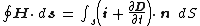# Displacement Current

Also found in: Wikipedia.

## Displacement current

The name given by J. C. Maxwell to the term ∂ D /∂t which must be added to the current density i to extend to time-varying fields the magnetostatic result of A. M. Ampère that i equals the curl of the magnetic intensity H . In integral form this result is given by the equation below,where the unit vector n is perpendicular to the surface dS. The concept of displacement current has important consequences for insulators and for free space where i vanishes. For conductors, however, the difference between the above equation and Ampère's result is negligible. See Maxwell's equations

If one defines current as a transport of charge, the term displacement current is certainly a misnomer when applied to a vacuum where no charges exist. If, however, current is defined in terms of the magnetic fields it produces, the expression is legitimate.

McGraw-Hill Concise Encyclopedia of Physics. © 2002 by The McGraw-Hill Companies, Inc.
The following article is from The Great Soviet Encyclopedia (1979). It might be outdated or ideologically biased.

## Current, Displacement

In establishing the theory of the electromagnetic field, J. C. Maxwell proposed a hypothesis— later confirmed by experiment—stating that a magnetic field is created not only by the motion of charges (conduction current, or simply current) but also by any change in the electric field over time. Maxwell designated as “displacement current” a quantity equal to the change over time t of the induction D—or, more accurately, the quantity (∂D/∂t)/4π. The rotational magnetic field is determined by the total current j = jcond + (∂D/∂t)/4π, where jcond is the conduction current density. A displacement current creates a magnetic field according to the same law that applies to a conduction current; it is for this reason that the designation “current” is applied to the quantity (∂D/∂t)/4π. (SeeMAXWELL’S EQUATIONS.)

The Great Soviet Encyclopedia, 3rd Edition (1970-1979). © 2010 The Gale Group, Inc. All rights reserved.

## displacement current

[dis′plās·mənt ‚kə·rənt]
(electromagnetism)
The rate of change of the electric displacement vector, which must be added to the current density to extend Ampère's law to the case of time-varying fields (meter-kilogram-second units). Also known as Maxwell's displacement current.
McGraw-Hill Dictionary of Scientific & Technical Terms, 6E, Copyright © 2003 by The McGraw-Hill Companies, Inc.
References in periodicals archive ?
From the initial moment and steady state, the total current in the first and second dielectrics is the same and has two components: active, which is caused by conduction current (leakage current), and reactive, due to displacement current (absorption current).
The effective electric current (i.e., displacement current) produced by the vacuum between the two metasurfaces can be defined and calculated as
(4) The MTL equations are written using a displacement current going through a capacitance and a true current following Kirchhoff's current law.
The next section explains frequency and waveform influence on the displacement current at the interface to approach IPMC behavior as a capacitor without a resistive element.
The conduction-current density [J.sub.[gamma]], the density of the external independent sources [J.sub.e] and the displacement current density [J.sub.D] has been related by the referring component of the electric vector-potential.
This work introduces the concept of an optical nanocircuit board, constituted of a layered metamaterial structure with low effective permittivity, over which specific traces that channel the optical displacement current may be carved out, allowing the optical "local connection" among "nonlocal" distant nanocircuit elements.
here c--proportion coefficient; E--electric field strength; [phi]--magnetic flux; H--magnetic field strength; I--conductivity current, proportional to the electric field strength [??]; [I.sub.S]--electric displacement current, proportional to [partial derivative] [??]/[partial derivative]t.
Which means that in metals we may neglect the displacement current as compared to the conduction one.
When nerve axon membranes are abruptly depolarized, a small outward displacement current precedes the sodium current that underlies the propagated nerve impulse.
Six flexible antennas mounted in the seat back cushion detect when a body enters the field because the displacement current changes.

Site: Follow: Share:
Open / Close